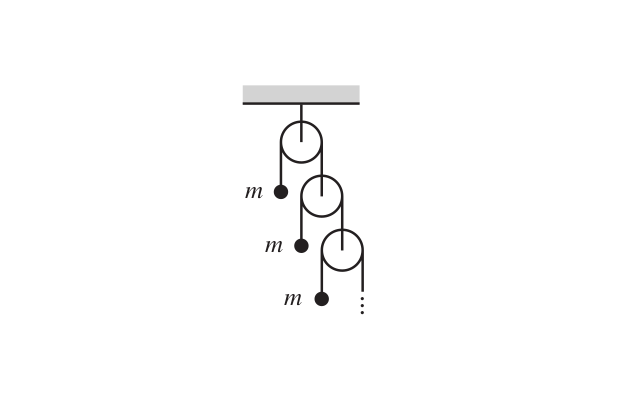The Infinite Atwood Machine

Consider the infinite Atwood’s machine shown in the figure.A string passes over each pulley, with one end attached to a mass and the other end attached to another pulley. All the masses are equal to m, and all the pulleys and strings are massless. The masses are held fixed and then simultaneously released. What is the acceleration of the top mass?

I have given the problem statement as it is. My humble request is for you all to help me obtain a mathematical solution to the above question rather than a trivial physical interpretation. Cheers!Note by Anirudh Chandramouli
3 years ago

MarkdownAppears as
*italics* or _italics_ italics
**bold** or __bold__ bold
- bulleted- list
• bulleted
• list
1. numbered2. list
1. numbered
2. list
Note: you must add a full line of space before and after lists for them to show up correctly
paragraph 1paragraph 2

paragraph 1

paragraph 2

[example link](https://brilliant.org)example link
> This is a quote
This is a quote
    # I indented these lines
# 4 spaces, and now they show
# up as a code block.

print "hello world"
# I indented these lines
# 4 spaces, and now they show
# up as a code block.

print "hello world"
MathAppears as
Remember to wrap math in $ ... $ or $ ... $ to ensure proper formatting.
2 \times 3 $2 \times 3$
2^{34} $2^{34}$
a_{i-1} $a_{i-1}$
\frac{2}{3} $\frac{2}{3}$
\sqrt{2} $\sqrt{2}$
\sum_{i=1}^3 $\sum_{i=1}^3$
\sin \theta $\sin \theta$
\boxed{123} $\boxed{123}$

Sort by:

Call the topmost block as $Block_0$, the next block as $Block_1$ and so on.

Let $a_i$ denote the acceleration (in the downward direction) of $Block_i$ for $i=0,1,2 \ldots$.

Then, by the principle of virtual work, we have: $\sum_{i=0}^{\infty} \frac{a_i}{2^i} = 0$

By Newton's second law, $mg - \frac{T}{2^i} = ma_i \quad i=0,1,2 \ldots$ Where $T$ is the tension in the string connecting $Block_0$.

Now, plugging in the value of $a_i$ (from the second equation), into the first one, and some simplification, we get $T = \frac{3}{2} mg$

Hence, $\boxed{a_0=-\frac{g}{2}}$

- 3 years ago

Where can I read about principle of virtual work? Btw , very nice & smart sol.n !,+1! @Deeparaj Bhat

- 3 years ago

I think the general notion of conservation of string is termed as principle of virtual work by some other concept of energy conservation, maybe? Am I right @Deeparaj Bhat ?

- 3 years ago

I believe in the latter. It is more commonly used than conplex words like "virtual work". Virtual work is nice but in real life it does not really apply because no machine is 100% efficient. Conservation of energy is better because it even covers dissipated energy.

- 3 years ago

Actually, the principle of virtual work is far more general. In fact, it's in the heart of Lagrangian mechanics.

But, no, it's not really very related to energy conservation.

- 3 years ago

Yeah, but I have used the latter term often.

- 3 years ago

yeah!! its more related to least action

i take it that u are invoking D'Alembert's form of the principle???

- 3 years ago

Yeah.

- 3 years ago

Why do you know lagrangian mechanics?:p

- 3 years ago

lol

i found this book by H.Goldstein in the basavangudi library which started with lagrangians and hamiltonian

- 3 years ago

Oh I'm reading that book as well. What a pleasant coincidence. Is it the one that starts with the mechanics of a single particle and then goes on to systems of particles and stuff?

- 3 years ago

That book was put in general by Julien in slack.

- 3 years ago

yes i know. that's how i got it...

- 3 years ago

Same here.

- 3 years ago

yes :)

- 3 years ago

But isn't the basis for Lagrangian mechanics that Euler Lagrangian formula for energy or whatever. That is why I assumed that it had to do with energy

- 3 years ago

It more or less comes to that. But, it kicks off with virtual work. After a lot of simplification (and assumptions), you get the form that you're talking about.

- 3 years ago

ok thanks anyways. I am new to Lagrangian mechanics and hope I will master it soon. :)

- 3 years ago

Are you all college guys!!

- 3 years ago

Nah I am in 11th :P

- 3 years ago

Just finished 12th :P

- 3 years ago

Hope so. @Swapnil Das , @Ashish Siva please comment.!

- 3 years ago

Yes both are there.

- 3 years ago

Umm. Kind of a mixture of both.

- 3 years ago

See this problem

- 3 years ago

I learnt from an old book of my dad's.

I don't know any good resource.

- 3 years ago

- 3 years ago

It is total work done is 0 in a fancy way

SUM Ti ai = 0

- 1 year, 6 months ago